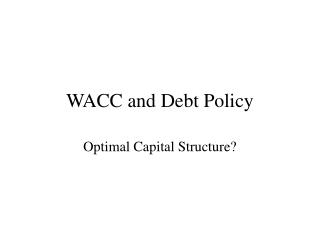# WACC and Debt Policy - PowerPoint PPT PresentationDownload PresentationWACC and Debt Policy

WACC and Debt Policy
Download Presentation## WACC and Debt Policy

- - - - - - - - - - - - - - - - - - - - - - - - - - - E N D - - - - - - - - - - - - - - - - - - - - - - - - - - -
##### Presentation Transcript

1. WACC and Debt Policy Optimal Capital Structure?

2. M&M (Debt Policy Doesn’t Matter) • Modigliani & Miller (Proposition I) • When there are no taxes and capital markets are perfect, the market value of a company does not depend on its capital structure. The Value of the firm does not change with debt: VL = VU

3. Return on Assets (wacc)No Taxes Note: rA = WACC (with no taxes)

4. M&M Proposition II The cost of equity capital increases with financial leverage – due to the increase in Risk! V = D + E These should be Market values!

5. M&M Proposition II r rE rA = WACC rD D E Risk free debt Risky debt

6. Leverage and Returns Impact on Beta

7. Leverage and Returns Impact on Beta If the Beta of Debt is assumed to be Zero BD = 0 The Beta of the Levered Firm is Equal to the Beta of the Unlevered Firm (or Asset Beta) times One plus the Debt-to-Equity Ratio Note: Equity betas are levered betas and asset betas are unlevered betas (L=E and U=A).

8. WACC (no taxes) • WACC is the traditional view of capital structure, risk and return.

9. Capital Structurewith taxes D x rD x Tc rD = D x Tc PV of Tax Shield = (assume perpetuity) Firm Value = Value of All Equity Firm + PV Tax Shield VL = VU + TC x D MM Proposition I with Corporate Taxes

10. MM Prop. I with Taxes Value of levered firm PV of interest tax shields Market Value of The Firm Value of unlevered firm Optimal amount of debt Debt

11. MM with Corporate Taxes MM Proposition II with Corporate Taxes r0 = the return on the all equity financed firm (the unlevered firm or the return on the assets of the firm)

12. Debt and Taxes Impact on Beta If the Beta of Debt is assumed to be Zero BD = 0 Remember, βL is the equity beta for a firm with leverage, and βU is the beta for the firm with NO debt

13. MM with Taxes: WACC r rE r0 WACC rD D V

14. Financial Distress Costs of Financial Distress - Costs arising from bankruptcy or distorted business decisions before bankruptcy. Market Value = Value if all Equity Financed + PV Tax Shield - PV Costs of Financial Distress

15. Financial Distress Maximum value of firm Costs of financial distress PV of interest tax shields Market Value of The Firm Value of levered firm Value of unlevered firm Optimal amount of debt Debt

16. WACC with Taxes Important: The WACC Formula

17. Weighted Average Cost of Capital (with costs of financial distress) r rE WACC rD D V Optimal amount of debt

18. Costs of Debt • Financial Distress Costs • Personal Tax Disadvantage of Debt • Agency Costs • Information Costs (or Benefits) of Debt • The Pecking Order Theory